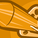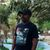# App Development

Discussion board where members can learn more about Qlik Sense App Development and Usage.

Announcements
April 22, 2PM EST: Learn about GeoOperations in Qlik Sense SaaS READ MORE
cancel
Showing results for
Search instead for
Did you mean:Contributor

## Rolling 3 month calculation for Days Sales Outstanding

Hi I am looking to calculate rolling average days sales outstanding.  The calculation would normally be:

Avg of previous 3 months AR

Avg of previous 3 months Revenue                                x 360

I am able to the formula to work for a single month but cannot get it to work as a rolling 3 months avg.  Below are the scripts that I am using for AR and revenue

//formula for Revenue for a single month

Sum({<[Exec P&L Heading]={"Revenue"},YEAR={'2018'},PERIOD={'<=\$(VCurrentMonth)'}>}MonthlyPostAMT)

//Formula for AR for a single month

Sum({<[BalanceSheetHeading]={"Accounts Receivable, net"},YEAR={'2018'},PERIOD={'<=\$(VCurrentMonth)'}>}MonthlyBalanceAMT)

also script for the variable VCurrent date is

=num(Month(today()),'00')

//v_CurrentDate

Any insight would be greatly appreciated.

Thanks!

1 Solution

Accepted SolutionsMVP

It is important that you use the right quote characters for literal strings/constant values and searches which should be single quotes for the former and double quotes for the latter.

Sum({<[Exec P&L Heading]={"Revenue"},YEAR={'2018'},PERIOD={'<=\$(VCurrentMonth)'}>}MonthlyPostAMT)

Should be:

Sum({<[Exec P&L Heading]={'Revenue'},YEAR={'2018'},PERIOD={"<=\$(VCurrentMonth)"}>}MonthlyPostAMT)

You are talking about the variable VCurrent month and showing the definition for v_CurrentDate (at least according to your comment) but in your expression you try to use a variable named VCurrentMonth ... is it just some typo?

Previous three months will not work properly in January and February if you reference YEAR and PERIOD. You should use a (calculated) rolling YEARMONTH field that can be created (if not already there) in your load script.

It could look like this:   201801, 201802, 201803 ....

To calculate it you could:

LOAD

.....

YEAR & Num(PERIOD,'00') AS YEARMONTH,

.....

Your expression would look more like:

Sum({<[Exec P&L Heading]={'Revenue'},YEAR={'2018'},PERIOD={">\$(v3MonthsAgo)<=\$(vCurrentMonth)"}>} MonthlyPostAMT)

vCurrentMonth:

=Date( Today(1) , 'YYYYMM')

v3MonthsAgo:

=Date( AddMonths(Today(1),-3) ,'YYYYMM')

For the average once you get the sum of the previous three months right you can only divide the sum by three of course...

2 RepliesMVP

It is important that you use the right quote characters for literal strings/constant values and searches which should be single quotes for the former and double quotes for the latter.

Sum({<[Exec P&L Heading]={"Revenue"},YEAR={'2018'},PERIOD={'<=\$(VCurrentMonth)'}>}MonthlyPostAMT)

Should be:

Sum({<[Exec P&L Heading]={'Revenue'},YEAR={'2018'},PERIOD={"<=\$(VCurrentMonth)"}>}MonthlyPostAMT)

You are talking about the variable VCurrent month and showing the definition for v_CurrentDate (at least according to your comment) but in your expression you try to use a variable named VCurrentMonth ... is it just some typo?

Previous three months will not work properly in January and February if you reference YEAR and PERIOD. You should use a (calculated) rolling YEARMONTH field that can be created (if not already there) in your load script.

It could look like this:   201801, 201802, 201803 ....

To calculate it you could:

LOAD

.....

YEAR & Num(PERIOD,'00') AS YEARMONTH,

.....

Your expression would look more like:

Sum({<[Exec P&L Heading]={'Revenue'},YEAR={'2018'},PERIOD={">\$(v3MonthsAgo)<=\$(vCurrentMonth)"}>} MonthlyPostAMT)

vCurrentMonth:

=Date( Today(1) , 'YYYYMM')

v3MonthsAgo:

=Date( AddMonths(Today(1),-3) ,'YYYYMM')

For the average once you get the sum of the previous three months right you can only divide the sum by three of course...Master

For previous 3 months try like this:

Sum({<[Exec P&L Heading]={"Revenue"},YEAR={'2018'},PERIOD={">=\$(=MonthStart(AddMonths(Max(PERIOD),-3)))<=\$(=MonthEnd(AddMonths(Max(PERIOD),-1)))"}>}MonthlyPostAMT)

/

Sum({<[BalanceSheetHeading]={"Accounts Receivable, net"},YEAR={'2018'},PERIOD={">=\$(=MonthStart(AddMonths(Max(PERIOD),-3)))<=\$(=MonthEnd(AddMonths(Max(PERIOD),-1)))"}>}MonthlyBalanceAMT)